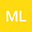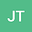3D structure - 2D plate - 1D rod interaction problem
••Josip Tambaca
University of Zagreb, Faculty of Science
Author Profile## Abstract

In this paper we consider the equilibrium problem of interaction of three elastic bodies of different elastic properties. The main body is the unit cube. On top of it is a thin layer/quboid of thickness $\eps$ of material whose stiffness is of order $\frac{1}{\eps}$ that in the middle contains another cuboid which is of width and thickness $\eps$ that is made of material with elasticity coefficients of order $\frac{1}{\eps^q}$ for $q>0$. We show that the family of solutions of linearized elasticity problems, when $\eps$ tends to zero, converges to a solution of a problem that is posed only on the unit cube with possibly additional elastic terms on the boundary related to the plate/rod energy of the thin elastic parts. It turns out that there are five different regimes related to different values of $q$ ($q\in \langle 0, 2\rangle, \{2\}, \langle 2, 4\rangle,\{4\},\langle 4, \infty\rangle$) with different limit problems. We further formulate a model posed on the unit cube that has the same asymptotics when $\eps$ tends to zero as the full 3d problem posed on the union of the unit cube and thin cuboids. This model then can be used as the approximating model in all regimes.
26 Aug 2022Submitted to Mathematical Methods in the Applied Sciences
27 Aug 2022Assigned to Editor
27 Aug 2022Submission Checks Completed
09 Sep 2022Reviewer(s) Assigned
21 Dec 2022Review(s) Completed, Editorial Evaluation Pending
22 Dec 2022Editorial Decision: Accept